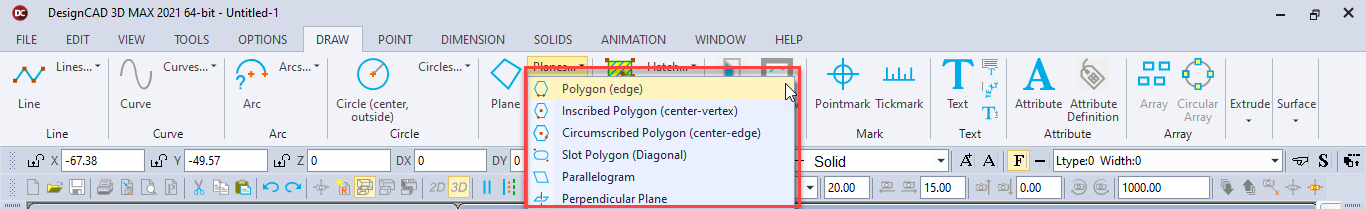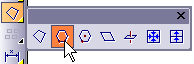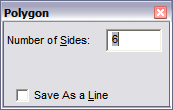# Polygon (Edge)Toolbox Icon:Draws a regular polygon - a convex shape in which each side is the same length. The number of sides can be entered in the window.

Point 1: Start of one side of the polygon

Point 2: End of one side of the polygon

Point 3: Orientation of the polygon (3D Mode only)

Enter the number of sides for the polygon in the

Number of Sides box.Set two points to designate one side of the polygon. If the drawing is in 3D Mode, a third point can be used to determine the plane on which the polygon lies.

If you select the Save as a Line option, the polygon will be saved as a line instead of a plane entity.

This command is similar to the Polygon (Center- Vertex) command, but with this command you set two points for one edge of the regular polygon.

With the Polygon (Center-Vertex) command, you set a point at the center and a point at a vertex or midpoint of a side of the polygon.

Example: Draw a polygon in your drawing.

Select the Polygon (Edge) command. Set a point for the beginning of one of the sides. Then move the cursor away from Point 1. A rubber-band polygon will be drawn using the cursor position as Point 2. When the polygon is the desired size, set Point 2. Hold down Ctrl while moving the mouse up or down and the polygon will swing on the axis of the first two points. When the polygon lies in the desired plane, set the last point.